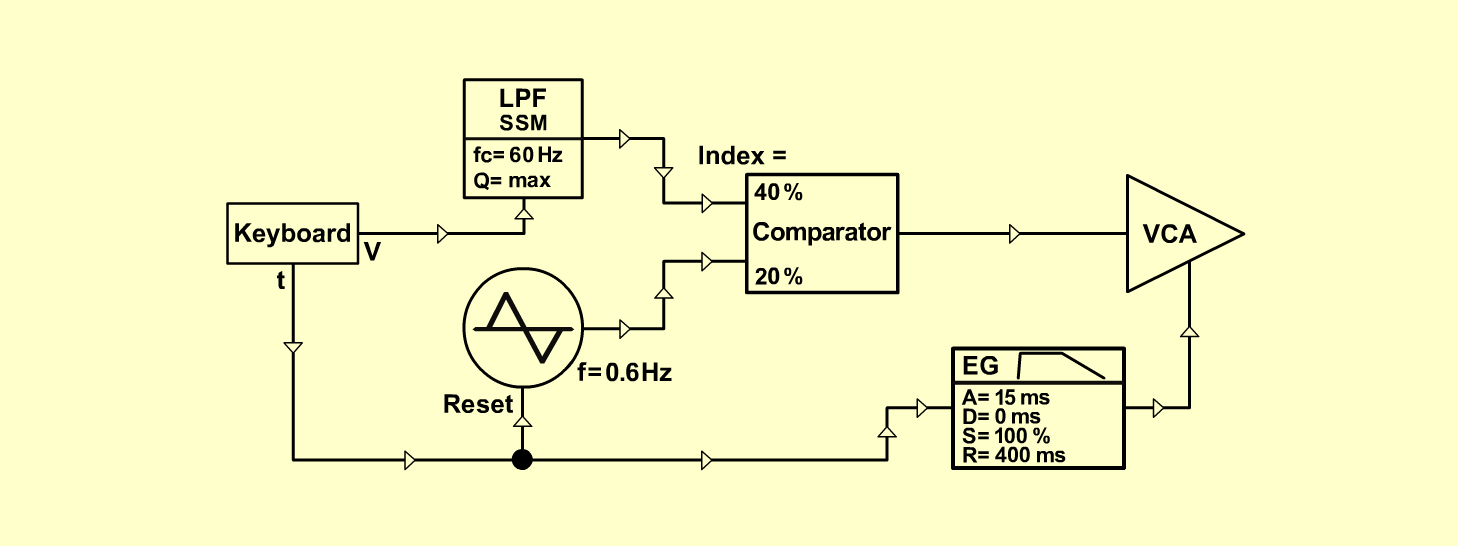Pwm-WaverS y n t hD o e p f e r A - 100 Connections: Settings: A-105 (Audio Out) <=> A-167 (+In)   A-145 (Triangle) <=> A-167 (-In)   A-167 (Cmp. Out) <=> A-131 (Audio In)   A-140 (Output) <=> A-131 (CV1)   A-131 (Audio Out) <=> Amplifier A-105 (Lev. = 10, Frq. = 3.75, Res. = 10)   A-131 (Gain = 0, Audio In 1 = 10, Audio Out = 10)   A-140 (A = 0.8, D = 7, S = 10, R = 6, Range = M)   A-145 (Frq = 2, Range = M)   A-167 (+In = 4, -In = 2, Offs. = 0, Gap = 0) Notes: Basic-patch for creating pulse-waves   out of filter-self-oscillation Ingo Zobel, August 2004   www.selfoscillate.de  *Sound samples Pwm-waver (65 Hz) Pwm-waver (130 Hz) Pwm-waver (261 Hz) Pwm-waver (523 Hz)